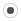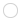This forum will soon be archived and you won't be able to interact with it anymore. Please use KDE Discuss instead.

## SVD of a complex matrixcpivot
Registered MemberPosts
1
Karma
0

### SVD of a complex matrix

Wed Oct 29, 2014 12:25 pm
Hello,
I'm try to perform the SVD of a complex matrix.
For exemple, this code :

Code: Select all
`#include <iostream>#include <complex>#include <Eigen/Dense>#include <Eigen/SVD>using namespace std;using namespace Eigen;int main(){   Matrix3cd A;   A << -2, -2, -9, -1, 1, -3, 1, 1, 4;   A(0,0)=complex<double>(-2,1);   cout << "Here is the matrix A:\n" << A << endl;   JacobiSVD<MatrixXcd> svd(A, ComputeFullU | ComputeFullV);   cout << "Its singular values are:" << endl << svd.singularValues() << endl;   cout << "Its left singular vectors are the columns of the thin U matrix:" << endl << svd.matrixU() << endl;   cout << "Its right singular vectors are the columns of the thin V matrix:" << endl << svd.matrixV() << endl;   cout << (svd.matrixU() * svd.singularValues().asDiagonal() * svd.matrixV().transpose() - A).norm() << endl;   }`

gives me this :
Code: Select all
`Here is the matrix A:(-2,1) (-2,0) (-9,0)(-1,0)  (1,0) (-3,0) (1,0)  (1,0)  (4,0)Its singular values are: 10.7767 1.641340.411582Its left singular vectors are the columns of the thin U matrix: (-0.879376,0.00595416)    (0.22305,-0.0956365)    (-0.350887,0.211288)(-0.270582,-0.00768811)    (-0.863744,0.422099)    (0.035271,0.0355187)  (0.391577,0.00747394)  (-0.0880244,0.0955685)    (-0.775269,0.478214)Its right singular vectors are the columns of the thin V matrix:   (0.225196,0.0819019)    (0.142557,-0.218302)     (0.249092,0.901418) (0.174428,-0.00112488)    (-0.851665,0.431929)   (-0.0928714,0.221481)(0.955067,-5.82171e-05)   (0.141158,-0.0141917)   (-0.118827,-0.231519)2.50259`

As you can notice, the norm of (USV*-A) is not close to 0.
If you change the third line of the code with A(0,0)=complex<double>(-2,0);
The norm is now : 3.02828e-15 and the result is OK compared to matlab.
I think that there is bug with SVD of a complex Matrix.

Do you have an idea to solve this problem?
Thank's
Byeggael
ModeratorPosts
3447
Karma
19
OS### Re: SVD of a complex matrix

Wed Oct 29, 2014 12:32 pm
You are taking the transpose of V instead of its adjoint. The correct test is:

cout << (svd.matrixU() * svd.singularValues().asDiagonal() * svd.matrixV().adjoint() - A).norm() << endl;

## Who is online

Registered users: asalan, Bing [Bot], Google [Bot], Sogou [Bot]